# Engineering Mechanics - Structural Analysis

### Exercise :: Structural Analysis - General Questions

• Structural Analysis - General Questions
6.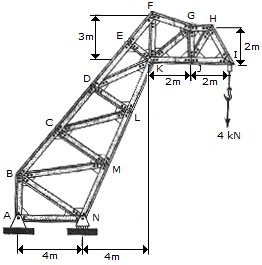A crane is constructed from two side trusses. If a load of 4 kN is suspended from one of these trusses as shown, determine the force in members FG, GK, and <KJ. State whether the members are in tension or compression. Assume the joints are pin-connected.

 A. KG = 2.66 kN C, KJ = 4.00 kN C, FG = 5.96 kN T B. KG = 5.66 kN T, KJ = 4.00 kN C, FG = 17.89 kN T C. KG = 0, KJ = 4.00 kN C, FG = 8.94 kN T D. KG = 1.886 kN C, KJ = 4.00 kN C, FG = 5.96 kN T

Explanation:

No answer description available for this question. Let us discuss.

7.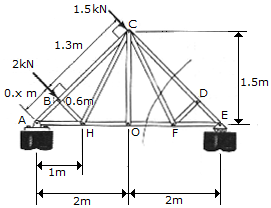Determine the force in members GF, CF, CD of the symmetric roof truss and indicate whether the members are in tension or compression.

 A. CD = 1.783 kN C, CF = 0, GF = 1.427 kN T B. CD = 3.48 kN C, CF = 00904 kN T, GF = 1.783 kN T C. CD = 2.79 kN C, CF = 0.723 kN T, GF = 1.427 kN T D. CD = 2.23 kN C, CF = 0, GF = 1.783 kN T

Explanation:

No answer description available for this question. Let us discuss.

8.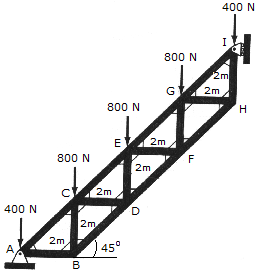The Warren truss is used to support a staircase. Determine the force in members CE, ED, and DF, and state whether the members are in tension or compression. Assume all joints are pinned.

 A. ED = 3.60 kN T, DF = 1.70 kN C, CE = 6.22 kN C B. ED = 2.00 kN C, DF = 2.26 kN C, CE = 2.26 kN T C. ED = 0.800 kN C, DF = 1.131 kN T, CE = 2.83 kN C D. ED = 0.400 kN C, DF = 2.26 kN T, CE = 4.53 kN C

Explanation:

No answer description available for this question. Let us discuss.

9.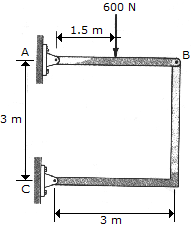Determine the horizontal and vertical components of force at pins A and C of the two-member frame.

 A. Ax = -212 N, Ay = 388 N, Cx = 212 N, Cy = 212 N B. Ax = -300 N, Ay = 300 N, Cx = 300 N, Cy = 300 N C. Ax = -849 N, Ay = 149 N, Cx = 849 N, Cy = 849 N D. Ax = -1200 N, Ay = 1200 N, Cx = 1200 N, Cy = -600 N

Explanation:

No answer description available for this question. Let us discuss.

10.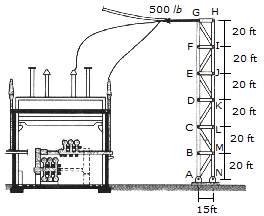A tower used in an electrical substation supports a power line which exerts a horizontal tension of T = 500 lb on each side truss of the tower as shown. Determine the force in members BC, CM, and LM of a side truss and indicate whether the members are in tension or compression.

 A. CM = 833 lb T, CB = 3330 lb C, LM = 2670 lb T B. CM = 581 lb T, CB = 2050 lb C, LM = 2000 lb T C. CM = 325 lb T, CB = 3040 lb C, LM = 2670 lb T D. CM = 58 lb T, CB = 1646 lb C, LM = 1600 lb T